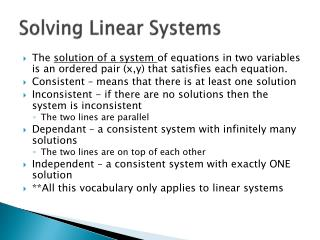DownloadDownload PresentationSolving Linear Systems

# Solving Linear Systems

Download Presentation## Solving Linear Systems

- - - - - - - - - - - - - - - - - - - - - - - - - - - E N D - - - - - - - - - - - - - - - - - - - - - - - - - - -
##### Presentation Transcript

1. Solving Linear Systems • The solution of a system of equations in two variables is an ordered pair (x,y) that satisfies each equation. • Consistent – means that there is at least one solution • Inconsistent - if there are no solutions then the system is inconsistent • The two lines are parallel • Dependant – a consistent system with infinitely many solutions • The two lines are on top of each other • Independent – a consistent system with exactly ONE solution • **All this vocabulary only applies to linear systems

2. Practice and Examples: • Graph each of the below and classify the system as consistent and independent; consistent and dependant or inconsistent: • Examples:

3. Steps: • 1) Solve for y (put in y=mx+b form) to make graphing easier • 2) Graph each line by using slope intercept form: • Find the y-int • Count off the slope • 3) Look for places that the graphs intersect and list these as solutions • 4) Verify solutions algebraically by plugging in each part of the ordered pair solutions

4. Solving Word Problems • Word Problems can be solved in the following manner • Create a linear model for the problem • Solve each linear equation for the same thing (what we do when we solve for y in the earlier problems) then set these equations equal to each other. • Examples: pg. 9 29 and 33

5. Finding Solutions • Algebraically – solve for one variable, set equal and find solution • Graphically – graph and look for intersection points • Simple Examples: • Solve: a) 2x=8 • b) x-5=2 • c) 2x+5=x-1

6. Solving Non-Linear Systems • Just like linear equations are solved by looking for the places that their graphs intersect; non-linear systems are also solved by finding intersection points. • Examples will be shown later….. • Solutions or intersection points can be found algebraically by setting each equation equal to each other. • Example – find the solution(s) for the below set of functions

7. Practice: • Find the solution(s) for the following set of functions algebraically:

8. Solving Non-Linear Systems • Example 1: • Use a graph to identify ordered pair solution(s) for the following set of equations

9. Graph should look like this: Identify Solutions Check Algebraically

10. Graph to find the solution for: • Example 2:

11. Solution is (0,-2)

12. Word Problem Practice Heather and Amanda each improved their yards by planting hostas and geraniums. They bought their supplies from the same store. Heather spent \$187 on 12 hostas and 13 geraniums. Amanda spent \$109 on 4 hostas and 11 geraniums. What is the cost of one hosta and the cost of one geranium?